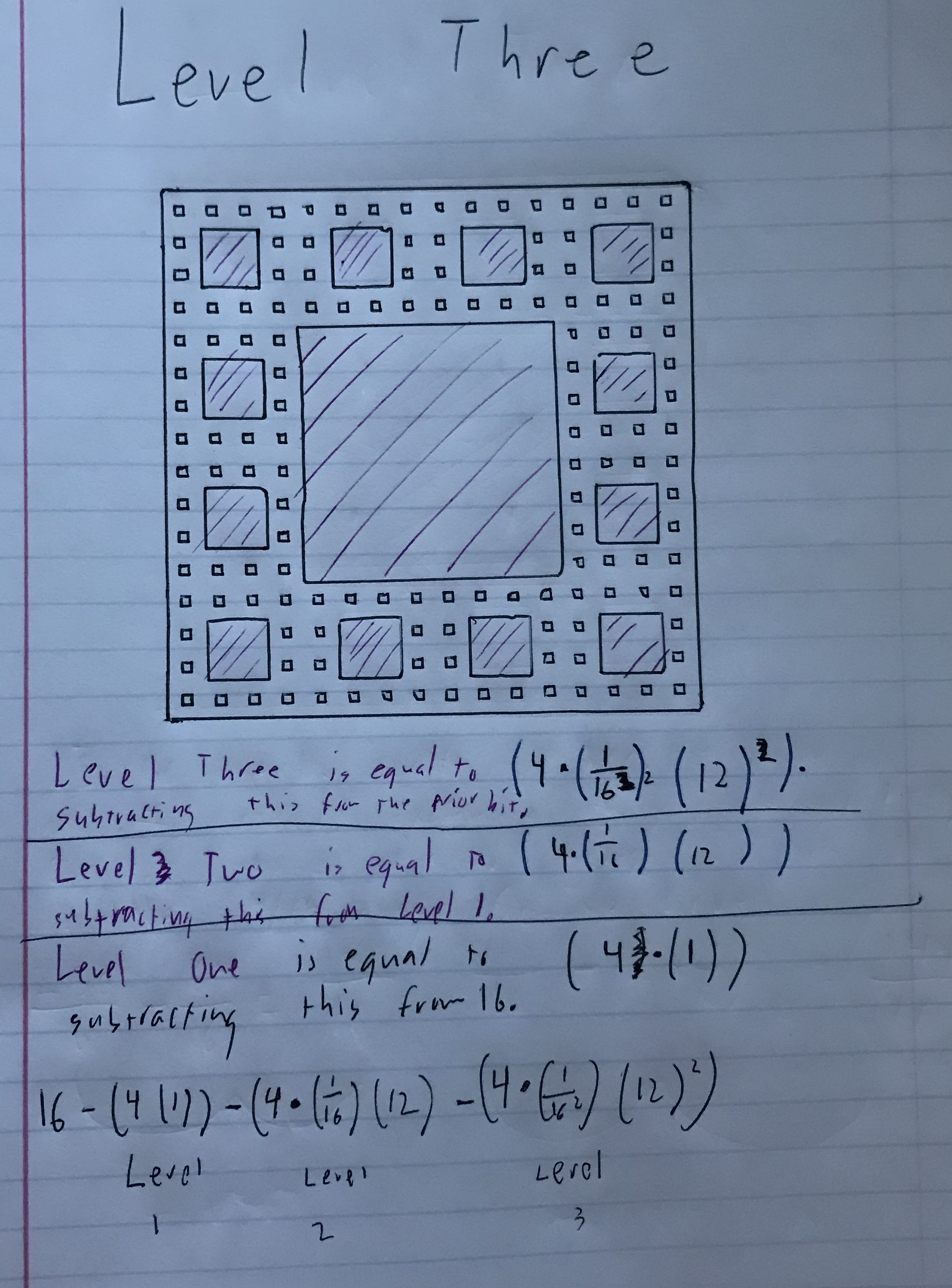# Centerless Carpet Fractal

For my Fractal Carpet, I removed the four center coordinates – (1,1) (2,1) (1,2) and (2,2).

This is what Level 1 of my Fractal Carpet looks like.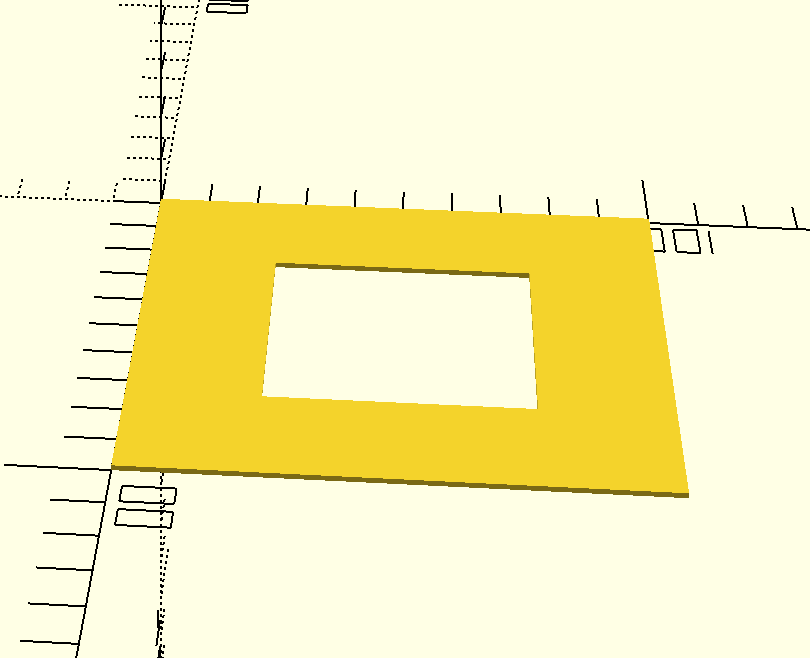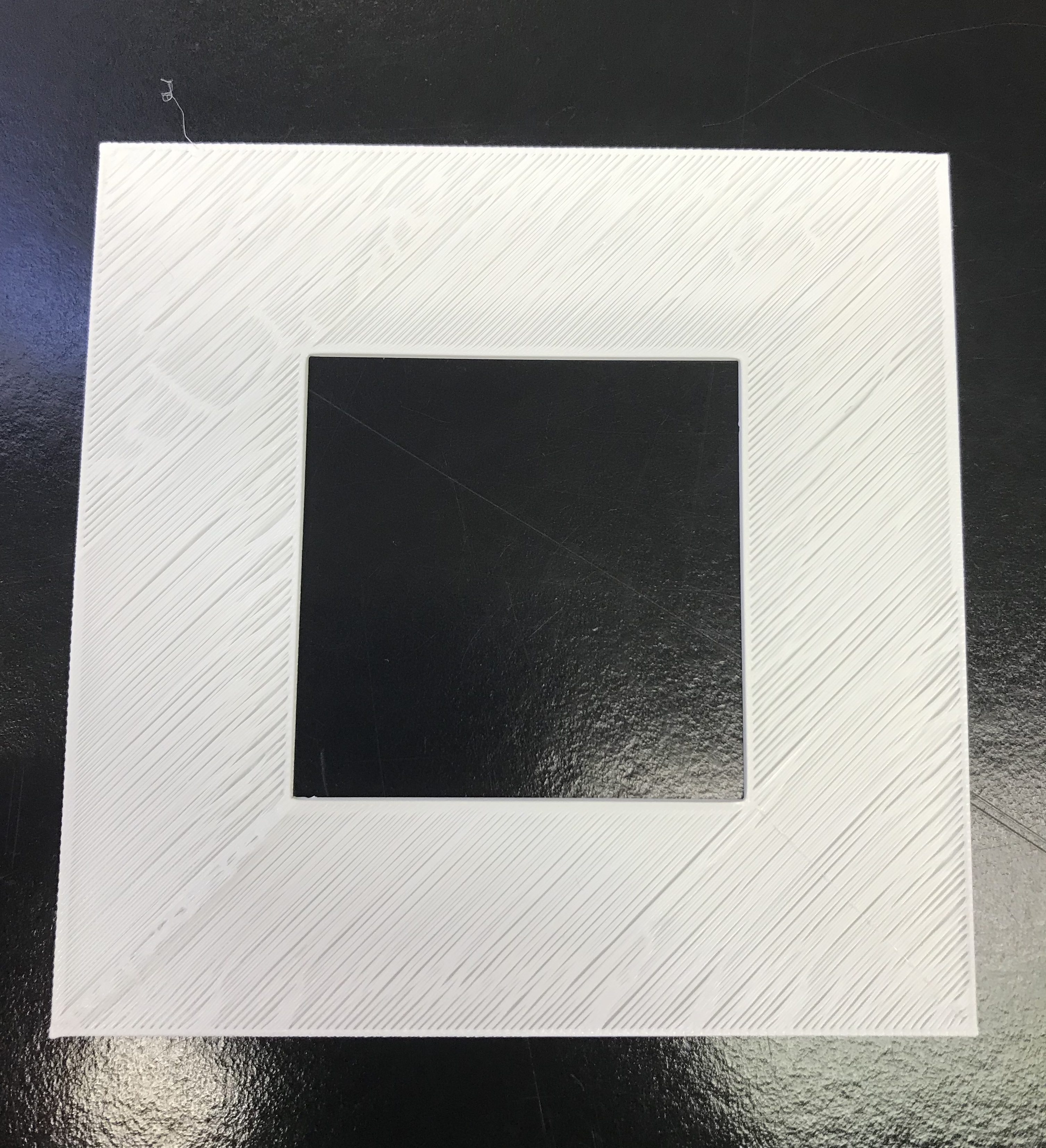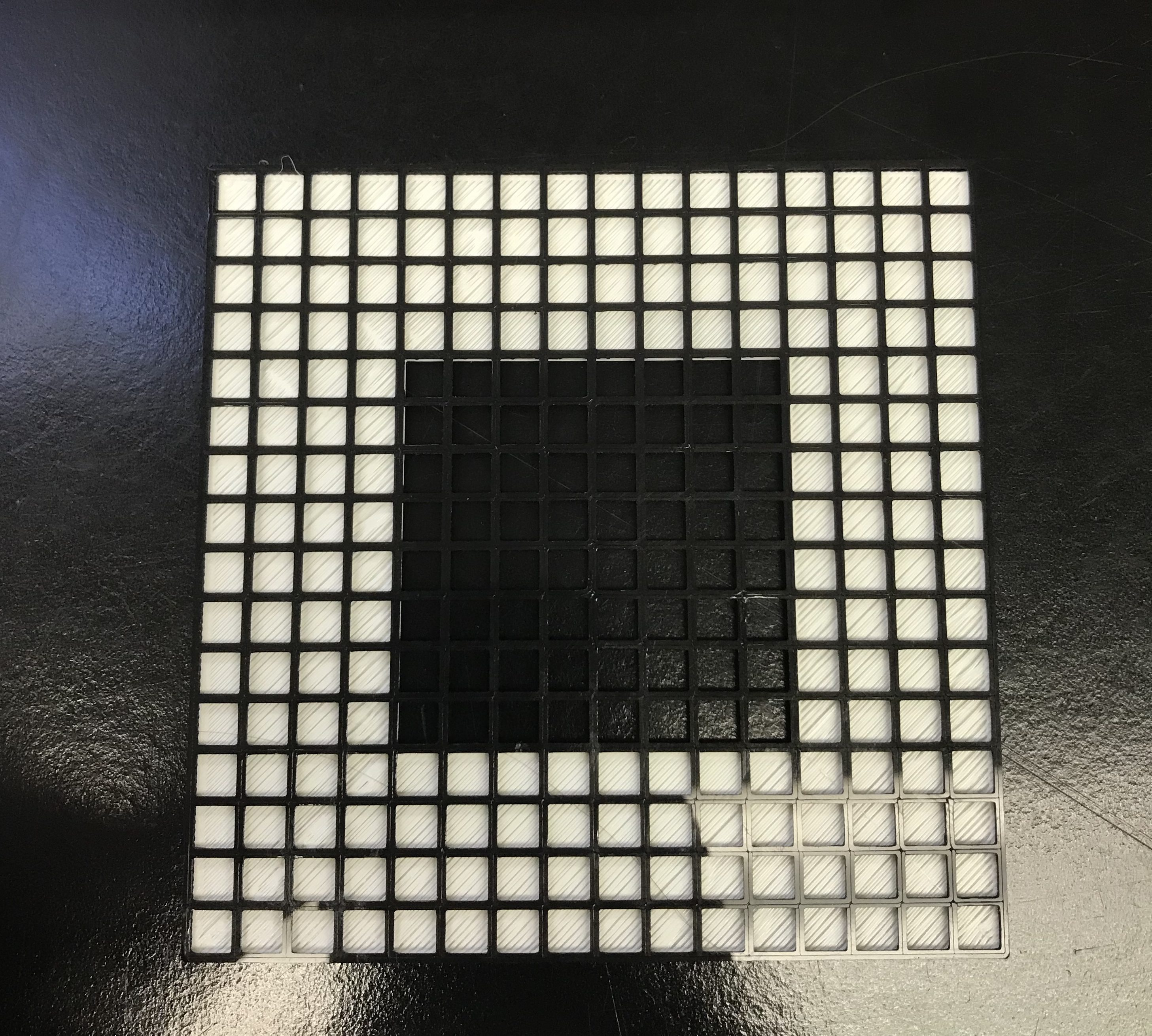The math for Level 1 is very simple. This Level has 16 equal units. I took out four. So 16 – 4 =12. The four in the center are the coordinates I took away.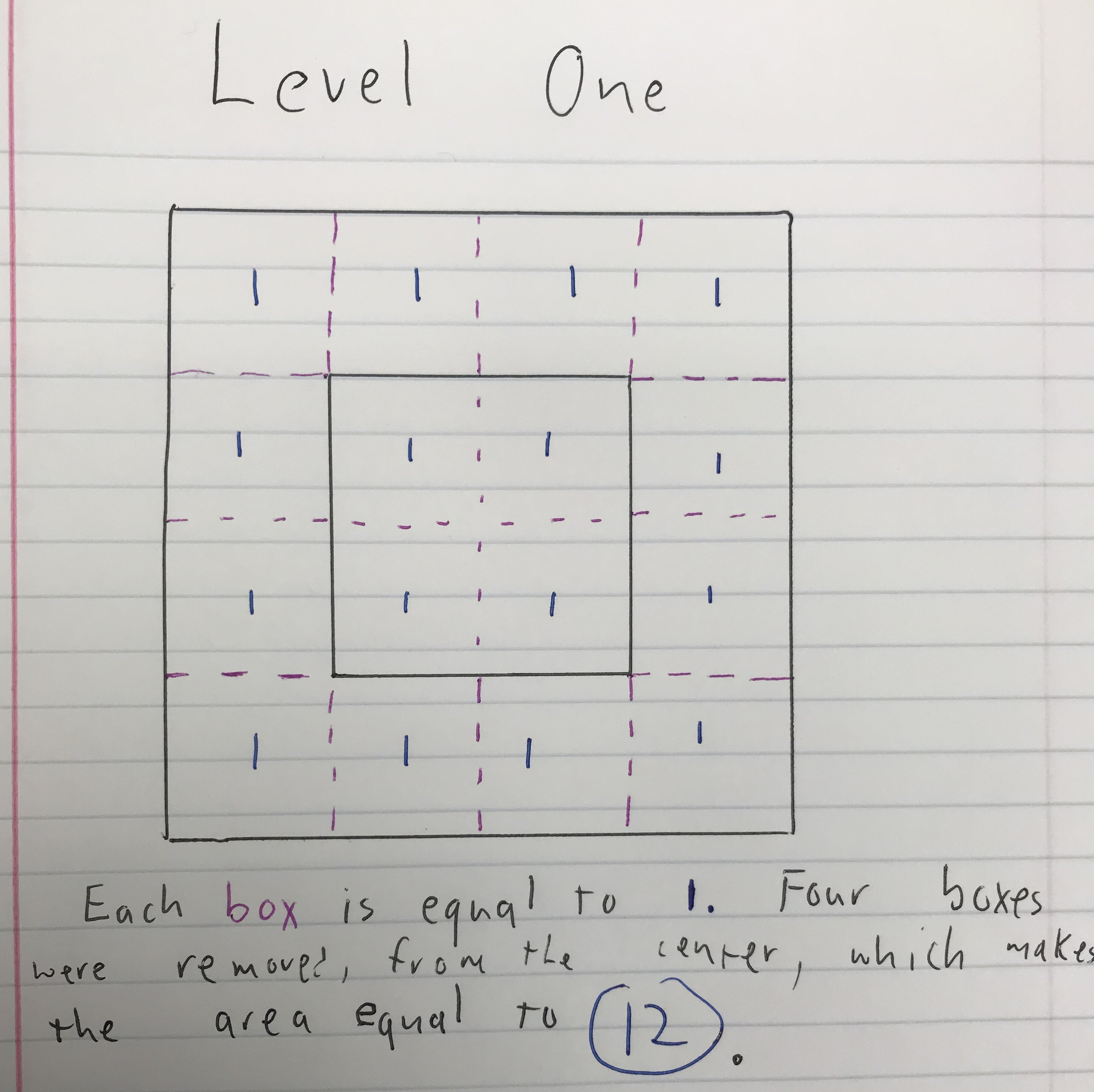The Level 2 iteration of my carpet looks like this.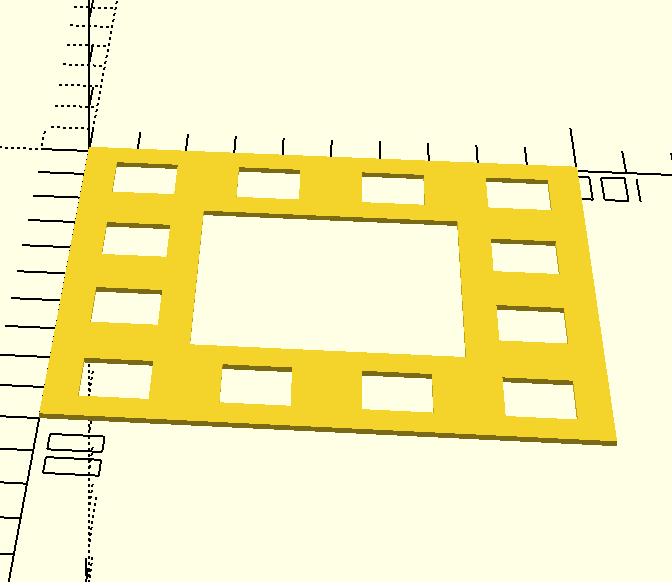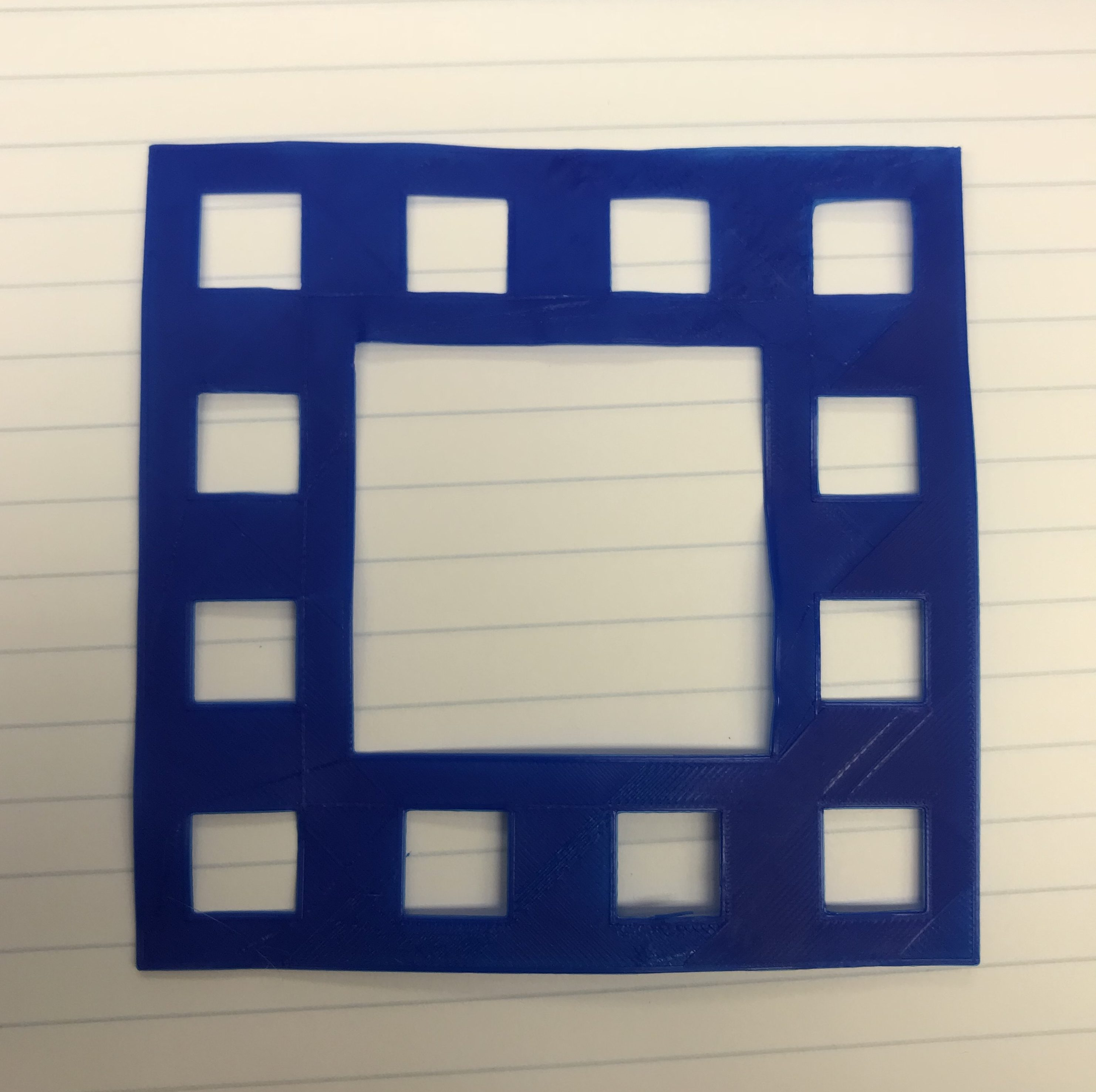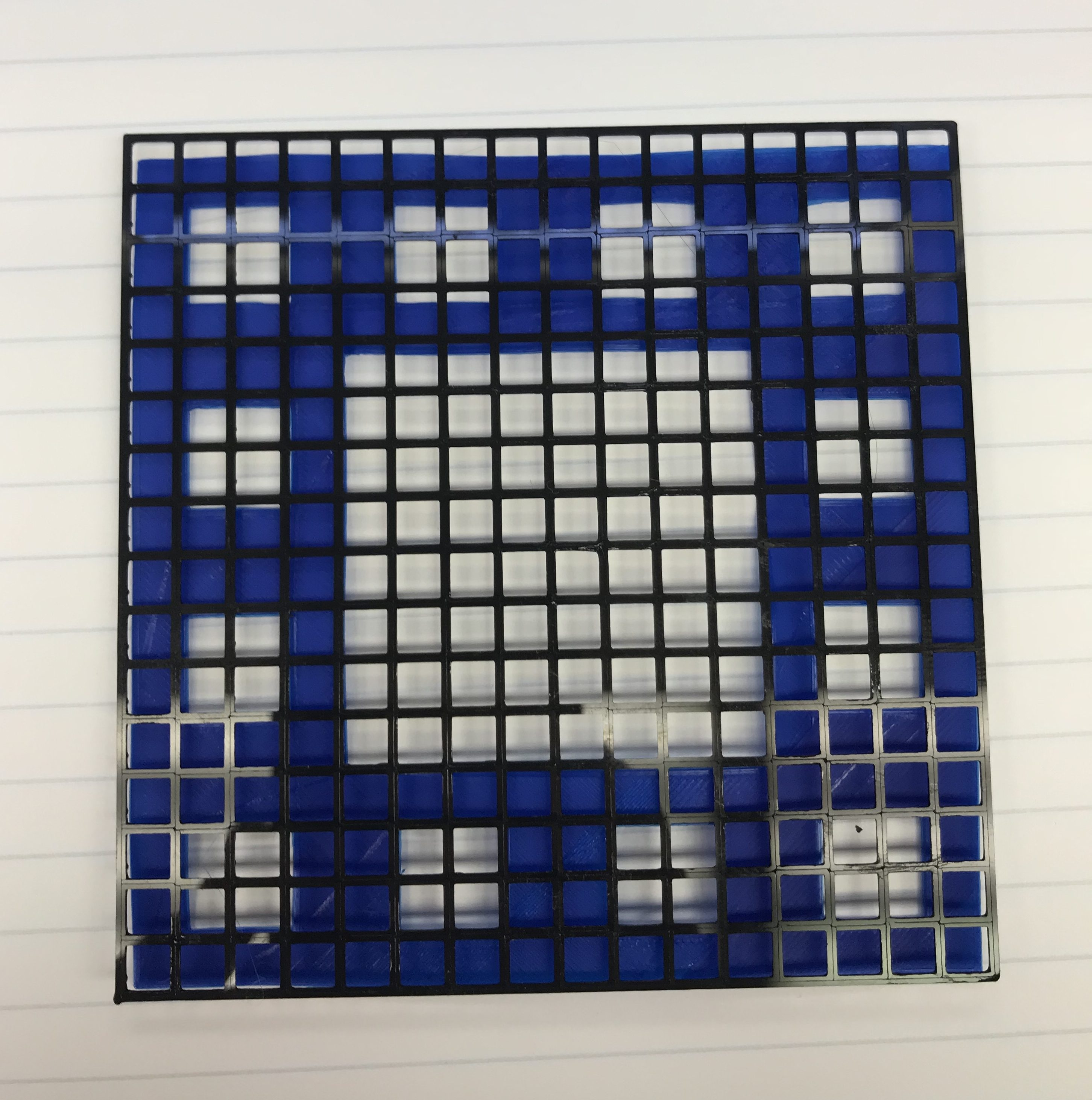I started with the area at 12, due to the fact the the four main center squares were removed. The second level created 12 new squares outside of the inner square. Each one of those squares has 16 small squares, and 1/4 of them were taken away. So from 12, we lost 1/4 or 4/16 of each square, which brings our area to 9, or 144/16. It is further explained below. (4 * (1/16)(12)).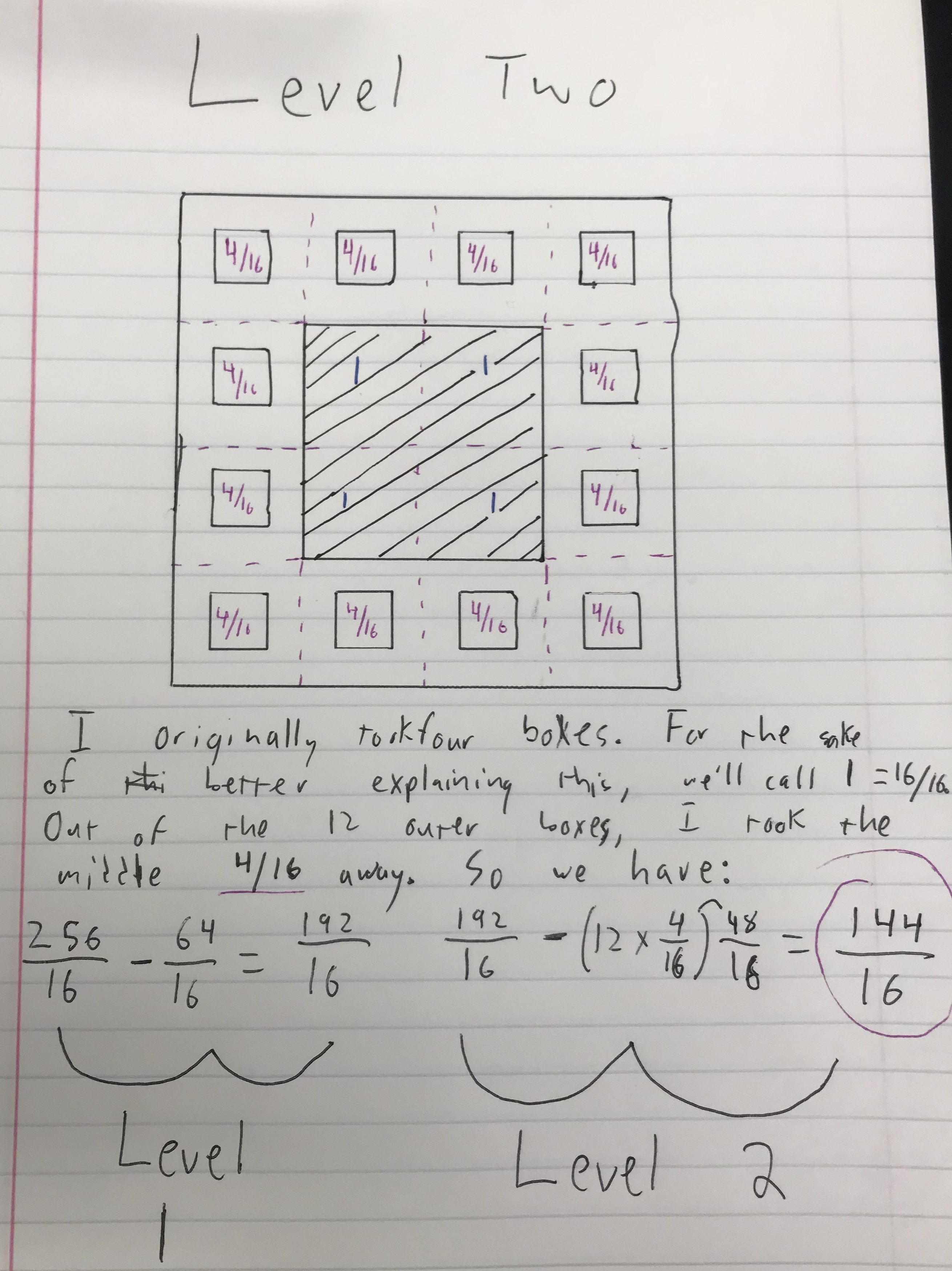The Level 3 iteration looks like this.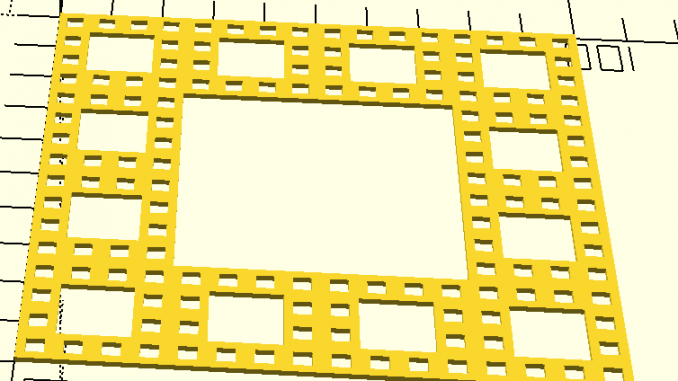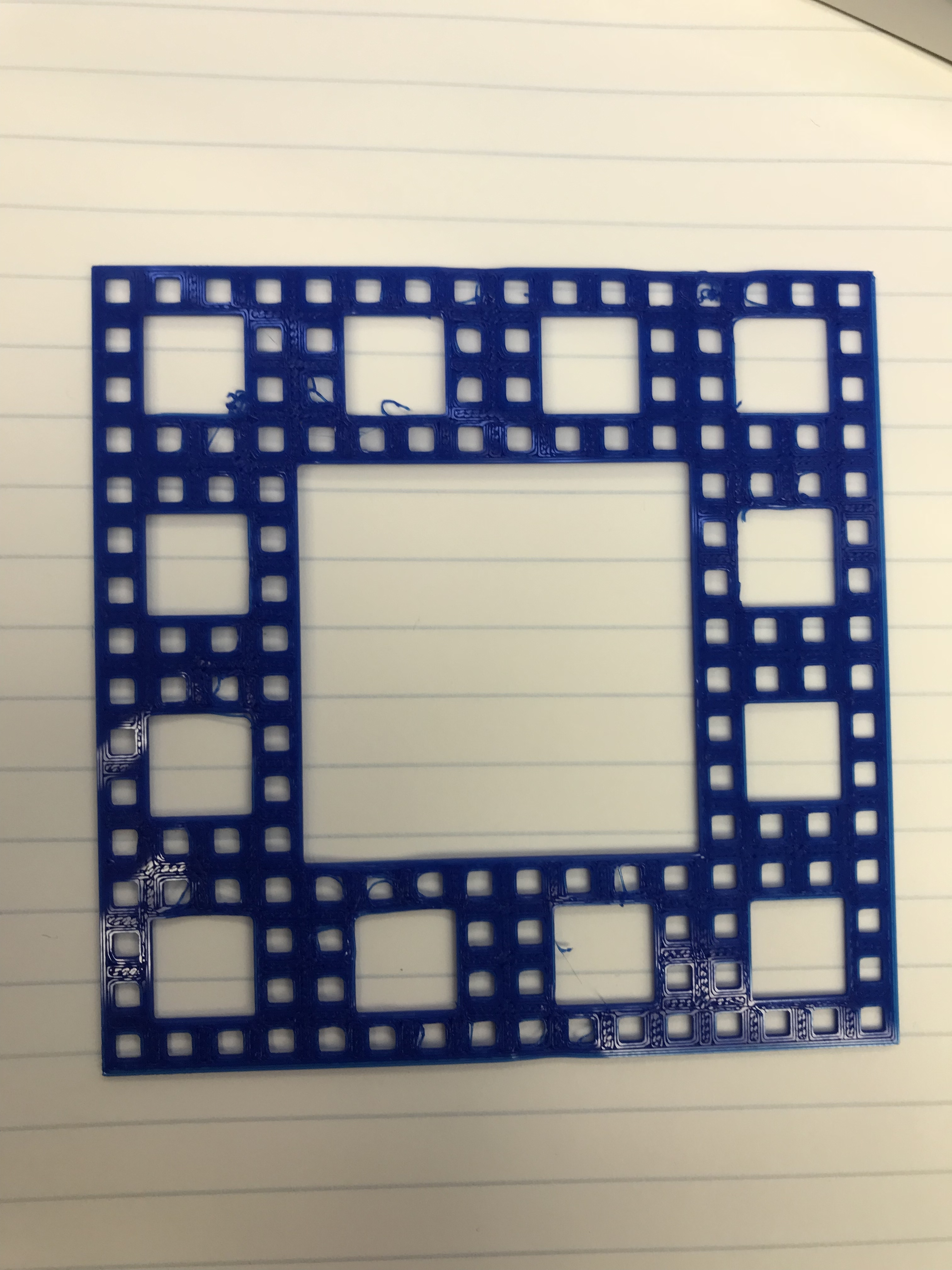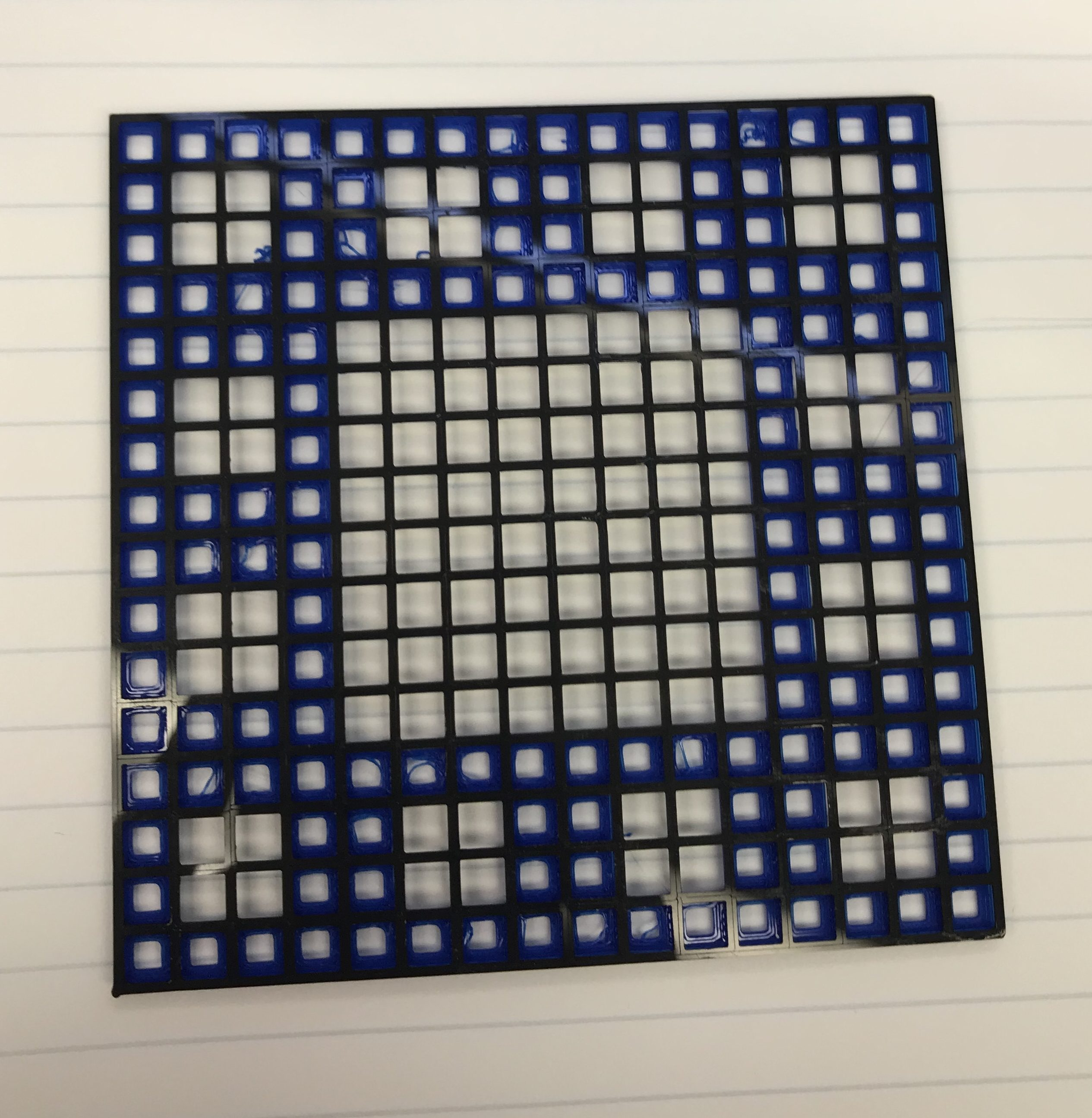Here is the calculation of the area of Level 3.